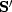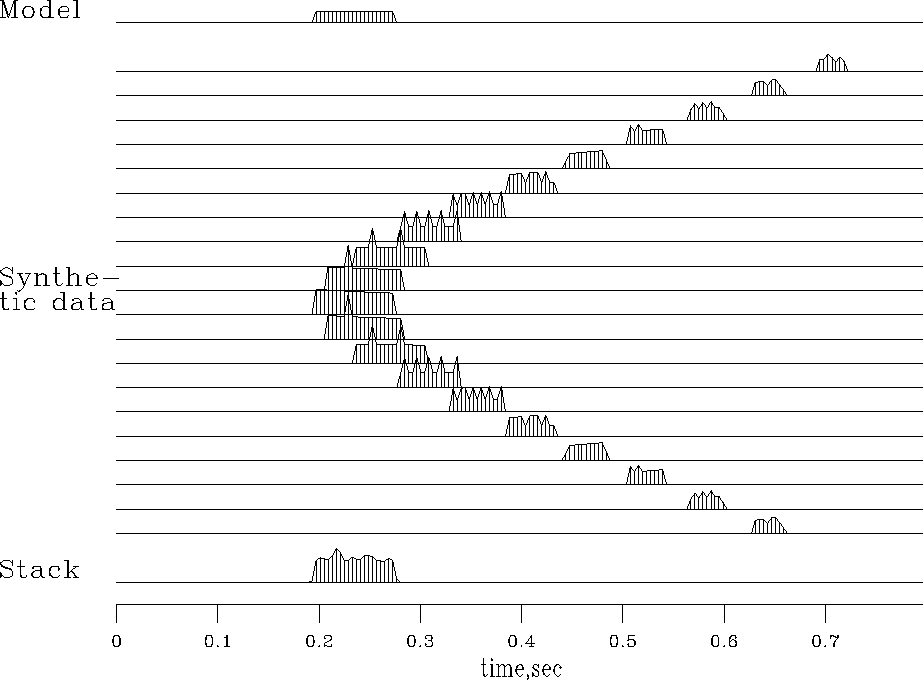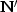Next: Pseudoinverse to nearest-neighbor NMO Up: NORMAL MOVEOUT AND OTHER Previous: Nearest-neighbor NMO

## Stack

Typically, many receivers record every shot. Each seismogram can be transformed by NMO and the results all added. This is called stacking'' or NMO stacking.'' The adjoint to this operation is to begin from a model that is identical to the near-offset trace and spray this trace to all offsets. There is no official'' definition of which operator of an operator pair is the operator itself and which is the adjoint. On the one hand, I like to think of the modeling operation itself as the operator. On the other hand, the industry machinery keeps churning away at many processes that have well-known names, so I often think of one of them as the operator. Industrial data-processing operators are typically adjoints to modeling operators.

Figure 6 illustrates the operator pair, consisting of spraying out a zero-offset trace (the model) to all offsets and the adjoint of the spraying, which is stacking. The moveout and stack operations are in subroutine stack1().

subroutine stack1( adj, add, slow,     t0,dt, x0,dx, nt,nx, stack, gather)
real     x,                  slow(nt), t0,dt, x0,dx,   stack(nt), gather(nt,nx)
do ix= 1, nx {
x = x0 + dx * (ix-1)
call nmo1( adj, 1, slow, x, t0,dt, nt, stack, gather(1,ix))
}
return; end

Letdenote NMO, and let the stack be defined by invoking stack1() with the conj=0 argument. Thenis the modeling operation defined by invoking stack1() with the conj=1 argument. Figure 6 illustrates both.

 stack Figure 5 Top is a model trace. Center shows the spraying to synthetic traces,. Bottom is the stack of the synthetic data,.Notice the roughness on the waveforms caused by different numbers of points landing in one place. Notice also the increase of AVO as the waveform gets compressed into a smaller space. Finally, notice that the stack is a little rough, but the energy is all in the desired time window.

We notice a contradiction of aspirations. On the one hand, an operator has smooth outputs if it loops over output space'' and finds its input where-ever it may. On the other hand, it is nice to have modeling and processing be exact adjoints of each other. Unfortunately, we cannot have both. If you loop over the output space of an operator, then the adjoint operator has a loop over input space and a consequent roughness of its output.

Unfortunately, the adjoint operatordefined by the subroutine nmo1()is not a good operator for seismogram modeling--notice the roughness of the synthetic seismograms in Figure 6. This roughness is not an inevitable consequence of nearest-neighbor interpolation. It is a consequence of defining the NMO program as a loop over the output space.Instead, we can define inverse NMO as a loop over its output space, which is notbut t. This is done in imo1().

subroutine imo1( adj, add, xs, t0, dt, nt, zz,     tt )
real                            t0, dt,    zz(nt), tt(nt),    t, xs, zsquared
do it= 1, nt {  t = t0 + dt*(it-1)
zsquared =  t * t - xs * xs
if ( zsquared >= 0.) {  iz = 1.5 + (sqrt( zsquared) - t0) /dt
if ( iz > 0 ) { if( adj == 0 )
tt(it) = tt(it) + zz(iz)
else
zz(iz) = zz(iz) + tt(it)
}
}
}
return; end


# inverse moveout and spray into a gather.
#
real xs,                       slow, x0,dx, t0,dt,    stack(nt), gather( nt,nx)